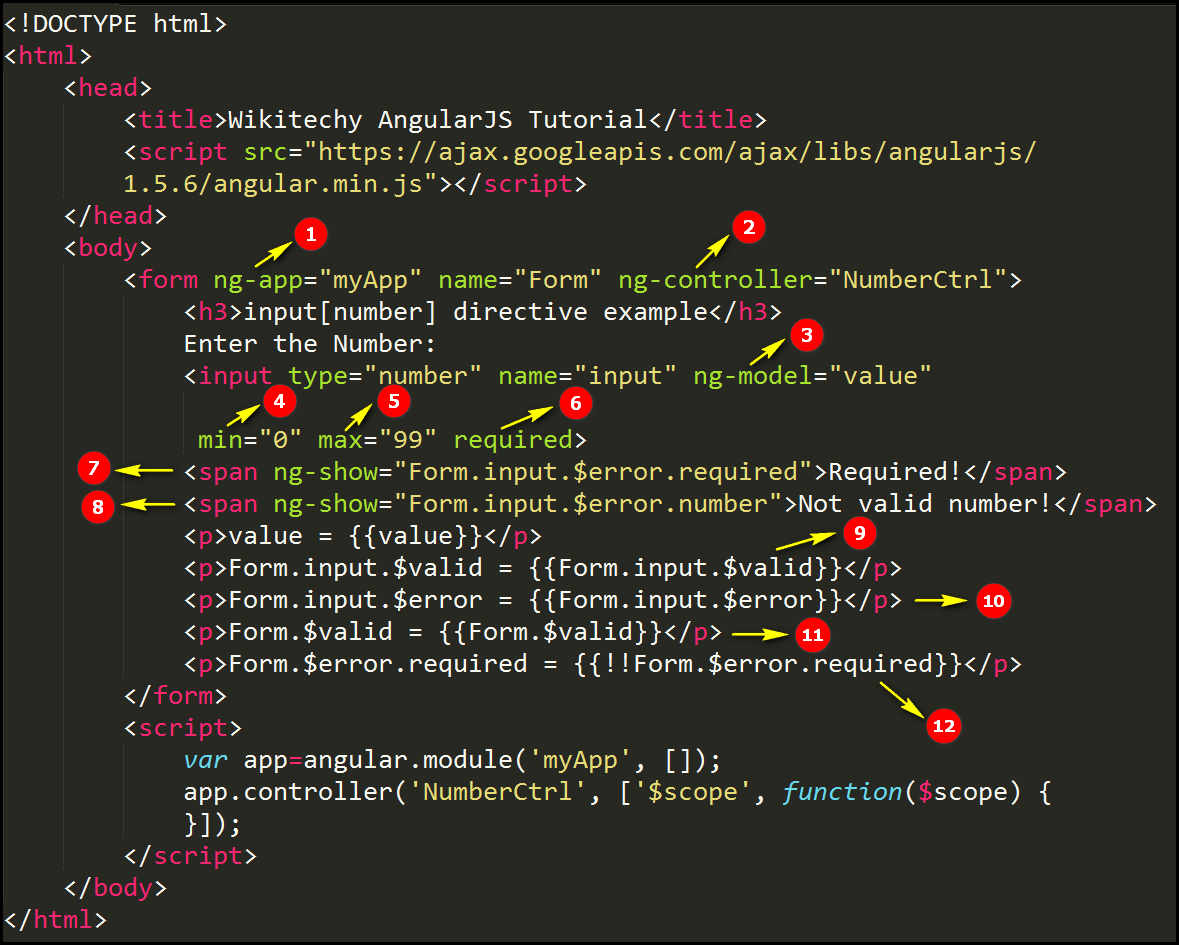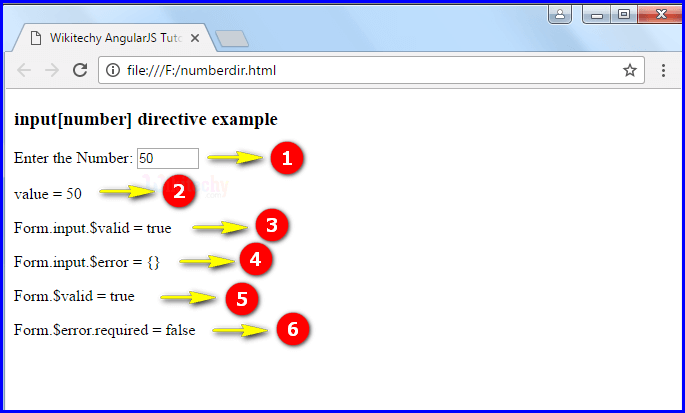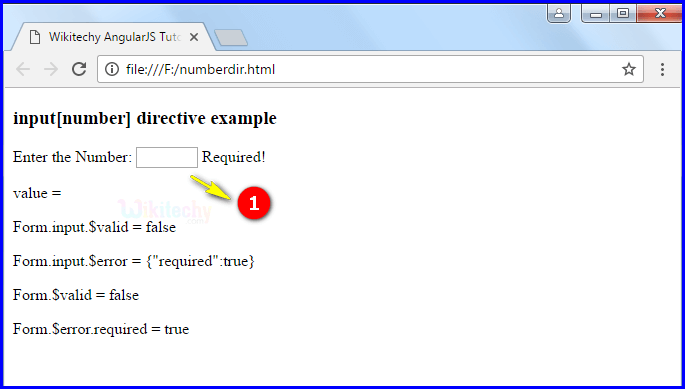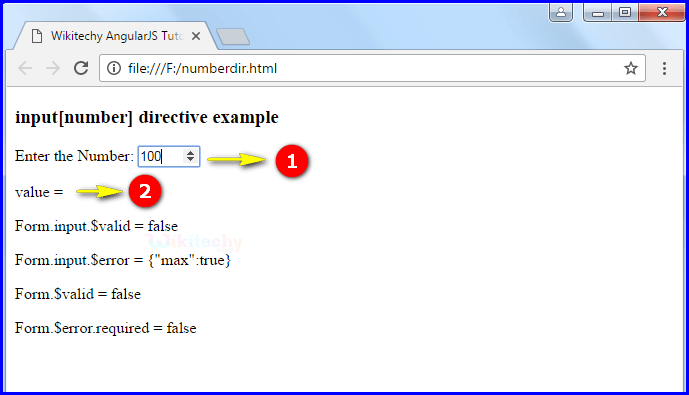# AngularJS Input Number

• input [number] is one of the AngularJS input directive in module ng.
• AngularJS directive input [number] is used to create an HTML text input with number validation and transformation.
• The input value must be a number otherwise error is thrown.
• This directive executes at priority level 0.

## Parameter Values:

Parameter Description
ngModel Defines angular expression to data-bind to.
name (optional) Name of the form under which the control is available.
min(optional) To set the min validation error key if the value entered is shorter than min.
max(optional) To set the max validation error key if the value entered is greater than max.
ngMin (optional) To set the min validation error key if the value entered is shorter than ngMin. It does not initiate HTML5 native validation. To Proceeds an expression.
ngMax (optional) To set the max validation error key if the value entered is greater than ngMax. It does not initiate HTML5 native validation. To Proceeds an expression.
step (optional) To sets the step validation error key if the value entered does not proper to the step constraint.
ngStep(optional) It same as the step. To sets the step validation error key if the value entered does not proper to the ngStep constraint. It does not initiate HTML5 native validation. To proceeds an expression.
required (optional) Denotes the required validation error key if the value is not entered.
ngRequired (optional) Sets the required attribute and required validation constraint to the element when the ngRequired expression sets to true.
Instead of required use ngRequired when we want data-bind to the required attribute.
ngMinlength (optional) States the minlength validation error key if the value is shorter than minlength.
ngMaxlength (optional) States the maxlength validation error key if the value is longer than maxlength.
pattern(optional) It is similar to ngPattern except that the attribute value is the actual string. It contains the regular expression body that will be converted to a regular expression as in the ngPattern directive.
ngPattern (optional) States pattern validation error key if the ng model value does not match RegExp found by evaluating the Angular expression given in the attribute value.
If the expression evaluates to a RegExp object, then this is used directly.
If the expression evaluates to a string, then it will be converted to a RegExp after wrapping it in ^ and \$ characters.
For instance, "abc" will be converted to new RegExp ('^abc\$').
ngChange (optional) An expression of Angular to be executed when input changes due to user interaction with the input element.

## Code Explanation input[number] directive in AngularJS:1. The ng-app specifies the root element (“myapp”) to define AngularJS application.
2. Ng-controller specifies the application controller in AngularJS the controller value is given as “NumberCtrl”.
3. The ng-model bind an input field value to AngularJS application variable (“value”).
4. min is used to set the minimum number starts from 0.
5. max parameter is used to declare the maximum number is 99.
6. If the number is not entered into the text field then the required parameter is used.
7. ng-show directive is used to hides HTML elements. If the required directive shows an error the content is displayed like “Required”
8. ng-show directive is used to hides HTML elements. If the required directive shows an error the content is displayed like “Not valid number”
9. Form.input.\$valid to checks the valid number or not. If the number placed between 0 to 99 then the output displays true otherwise false.
10. Form.input.\$error to check whether the valid number or not .If the number specified in error it through the exception (Like “required”=”true) otherwise it is an empty curly braces ( { } ).
11. Form.\$valid is used to check whether the form is valid or not and output will be displayed in <p> tag.
12. Form.\$error.required is used to number is required or not. If the number is required and output will be displayed as false otherwise true.

## Sample Output input[number] directive in AngularJS:1. If the user scroll the month in the input field.
2. The output will be displays as value=50.
3. The output displays true because it is consider as a number from 0-99.
4. The output displays empty curly braces it means does not thrown any error.
5. The output displays true it is valid month.
6. If the text box does not empty so the output displays as false.1. If user does not specify any month in the input field and output displays as “Required”.1. If user specify the number is 100 in the input text field. (The value starts from 0-99)
2. The output displays value is an empty.